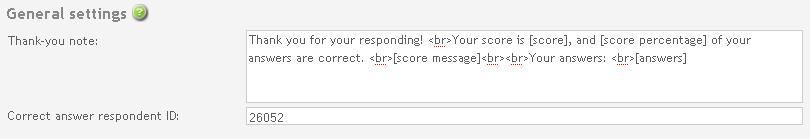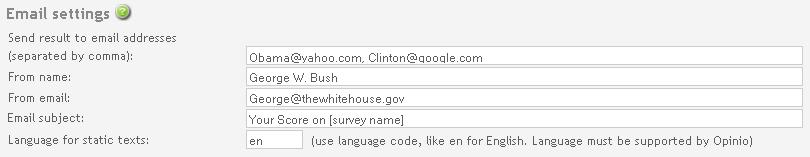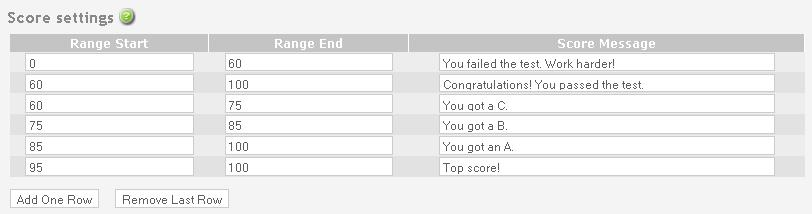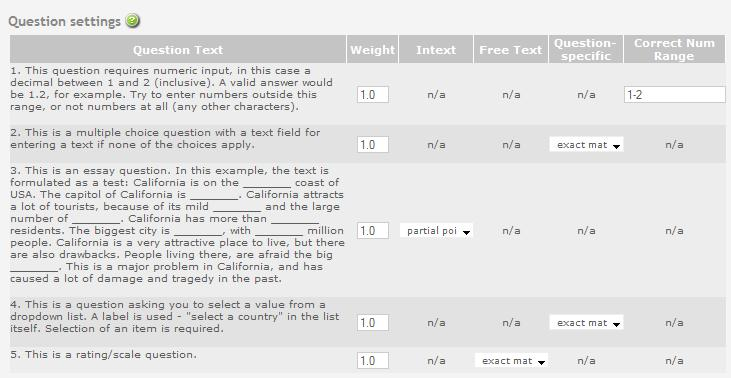# Quiz Plugin Help

## Table of Contents

### Introduction

When a respondent completes a survey, the Quiz-plugin computes a score based on the number of correct answers, and the importance (weight) of each question. Survey administrator will be able to define the question weights and score ranges, as well as provide the correct respondent ID (where the "correct answers" can be found) within the setup page. In addition to a score, a detailed report on the responses to all questions will be generated and displayed in the "thank-you note", and optionally sent by email to the survey administrator or other email recipients.

To activate this plugin, check the "Activate plugin" checkbox at the top of the page. Note: all of the existing settings will be lost if you uncheck (deactivate) the plugin.

After filling in the set up values, remember to click the "Save" button at the bottom of the page to save the settings. If the structure of the survey has been changed since you entered this setup page (e.g. some of the questions are modified, added, deleted or moved), when the "Save" button is clicked, this plugin will show a message indicating this change, and ask you to verify/modify the settings accordingly.

### General settings

A customizable "thank-you note" and the respondent ID with correct answers can be configured in this section. Both items are required.#### Thank-you note

The "thank-you note" will be shown to the respondent when the survey/quiz is completed. This message can be customized according to your preference. The tags [survey name], [score], [score percentage], [score message] and [answers] can be used to place the survey name, score, percentage of correct answers, score message and the the respondent's responses respectively at the desired place in the message. If for example you do not want to show the detailed report on the responses to each question, then omit the [answers] tag.

#### Correct answer respondent ID

This is the respondent ID that contains correct and standard responses to the survey. The responses from other respondents will be compared with this respondent's answers. The administrator is responsible for providing correct answers to the survey/quiz in beforehand, and filling in the correct respondent ID here. The respondent ID can be found via "Reports and data" -> "Manage responses".

### Email settings

Optionally, the results of the quiz responses can be sent by email to the administrator or other email recipients. If you decide to send results by email, then all the fields in this section must be filled in.#### Send result to email addresses

Fill in the email addresses where the quiz results can be sent. If there are more than one email addresses, these addresses should be separated by commas.

#### From name

This is the "from name" that will appear in the "from" field when the recipients receive the email.

#### From email

This is the "from email" that will appear in the "from" field when the recipients receive the email.

#### Email subject

This is the subject message that will appear in the "subject" field when the recipients receive the email.

#### Language for static texts

This is the language code (en for English) used to show static texts in the email. The Language must be supported by Opinio.

### Score settings

Score ranges can be set up in this section. The ranges should be from the lowest to the highest, and start from 0 in the first range. It is allowed to enter ranges that intersect each other. In that case, if a score falls into the intersected value ranges, then all the score messages that apply will be shown in the report message.
The "Add one row" and "Remove last row" buttons can be used add or delete rows in the range table.#### Range start

The starting point of the score in the specified range. This starting value is inclusive ONLY in the first range (which is the lowest range), and exclusive in all the other ranges.

#### Range end

The ending point of the score in the specified range. This ending value is inclusive in the range.

#### Score message

The score message will be shown if the respondent's total score falls into this particular range.

### Question settings

This section sets up how the scores are computed for the questions within the survey/quiz. For each individual question, the survey administrator can set up the question weight, choices for intext and free text (if they exist), and other question-specific settings.#### Weight

This is the place to set up the question weight, which could be an integer or a floating point number.

#### Intext

This determines how the scores of intext items are computed. The default choice is "partial points", which means the question, including all the intext items sum up to one point; If "one point per item" is chosen, each correct intext item is worth one point.

#### Free Text

This determines how the score of the free text field is computed. There are 4 choices, the default choice is "exact match", which means that the respondent's answer should match the correct answer exactly (not case sensitive) to obtain points; With "at least one", if the answer matches one of the correct answers, it is considered correct; Furthermore, there are "exact match - match adds one point" and "at least one - match adds one point", which basically have the same meaning as its corresponding choice above, except that correct free text response is worth one extra point.
If "at least one" or "at least one - match adds one point" is selected, the correct answers should be separated by commas in the free text field of the correct response (belonging to the respondent specified by the "correct answer respondent ID").

#### Question-specific

If the question is one of the following three types: (1) multiple choice question (with multiple selection allowed), (2)dropdown question (with multiple selection allowed), or (3) matrix question, the "Question-specific" options will be available.
For a multiple choice question, there are two choices available: if "exact match - multiple choice" is selected, then the point is gained only if the response matches the correct answer exactly, including the "other" field if it exists; with "partial match - multiple choice" however, the response can be "partially correct" and thus obtains partial score points, i.e, as long as the response contains (part of) the correct options, the corresponding scores are obtained. Note it is up to the quiz designer to control that the size of response options is the same as the correct answer.
For a dropdown question, like multiple choice questions, there are also two choices available: "exact match - dropdown list" says only if the response matches the correct answer exactly, the score point is obtained; With "partial match - dropdown list", a partially correct answer will obtain partial score.
For a matrix question, if the default choice of "matrix - partial points" is selected, each correct items in the matrix will obtain a partial point, and all the items within the matrix sum up to one point; if "matrix - one point per item" is selected, then each correct response item of the matrix is worth one point.

#### Correct Num Range

If the corresponding question is of type numeric, in addition to providing a correct numeric value in the standard respondent answers, a range of correct values can be filled in here. If an answer to the question falls into one of the specified ranges, it is considered correct.
Valid value ranges could be like:
5.8-6.9, 8, 16.5-18
There can be both value ranges (such as "5.8-6.9", starting and ending values are inclusive) and individual values (such as "8"). Value ranges should be separated by commas, as shown in the above example.

#### Examples on score computations

The total score of each question equals to the total points of the question (including intext and free text fields if they exist), times the question weight:  score = points * weight

The default weight of an individual question is 1.0, and the whole question, including possible intext fields and free text field, shares 1 point by default. Thus, if the responses to the whole question are totally correct, the respondent will gain a score of 1.0. However, this can be adjusted by the choices described above, so that a matrix item, an intext item, or free text obtains a separate point.

Consider the following examples:

A rating question has 6 intext items and a free text field. If the question weight is set to 2.0, and both "one point per item" in intext setting and "exact match - match adds one point" in free text setting are selected, the "totally correct" answers to this question would then give a score of 16.0:
score = ( 1 + 6 + 1 ) * 2.0 = 16.0
The first "1" point is for the question itself, "6" points for intext items, and the second "1" point for free text.

On another occasion, for the same question, if "partial points" in intext setting and "exact match - match adds one point" in free text setting are selected, the "totally correct" answer would then give a score of 4.0:
score = ( 1 + 1 ) * 2.0 = 4.0
The first "1" point is for the question and intext items combined, while the second "1" point for free text; A correct answer to an intext item will thus obtain one seventh of a point.

Finally, if the question weight is 1.0 (default value), and "partial points" in intext setting and "exact match" in free text setting are selected, the "totally correct" answer to the whole question would give a score of 1.0:
score = 1 * 1.0 = 1.0
i.e. the whole question equals "1" point; A correct answer to an intext item will thus obtain one eighth point. A correct rating answer will also obtain one eighth point.